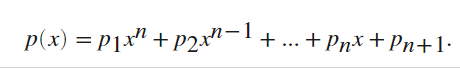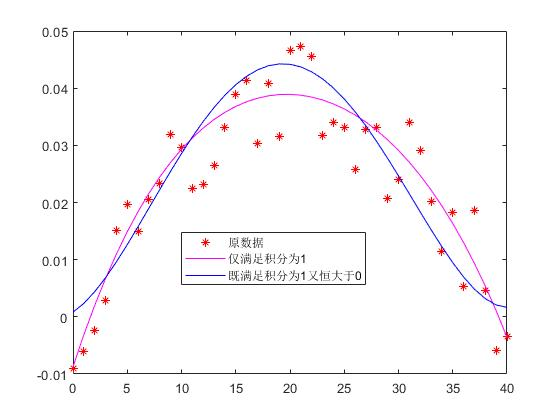2021-10-01 23:27

matlab中进行函数拟合，如何同时添加等式和不等式两种约束条件H=histogram(X,'Normalization','probability');
x = (H.BinEdges(1:end-1)+H.BinEdges(2:end))/2;%风速
y = H.Values; %这就是对应的频率
n=10 %设n
x = x(:);
y = y(:);
S = zeros(length(x), n+1);
q = ones(length(x),1);
S(:,n+1)=q;
for i = n:-1:1
q = q.*x;
S(:,i) = q;
end
A = S'*S;
b = S'*y;
a = A\b;%最小二乘
G = 1./(n+1:-1:1).*40.^(n+1:-1:1); % 积分为1
aa = [A, G'; G, 0]\[b;1];%拉格朗日乘子法
y1=polyval(a,x)
y2=polyval(aa(1:end-1),x)
plot(x,y1)
hold on
plot(x,y2)
legend('原始风速数据','拟合后(不考虑积分为1)','拟合后(考虑积分为1)')

• 写回答
• 好问题 提建议
• 追加酬金
• 关注问题
• 收藏
• 邀请回答

3条回答默认 最新

•joel_1993 2021-10-07 16:35
已采纳

你好，之前也提醒过题主用fmincon函数，现在把程序发一下，我是按照fmincon求解的（额外加了非线性约束保证函数值全体大于0），但是初值选取使用的是拉格朗日乘子法加最小二乘法【这样计算比较稳定】。代码如下

function main
x = [0:1:40]; % x自己设置
y = pi/80*sin(1/40*pi*x)+(rand(size(x))-0.5)*pi/80*1/2;% y自己设置，我这里是在正弦函数上加了个随机浮动
n = 4; % n自己设置
f = @(a)norm(polyval(a,x)-y);
aa = getIniA(x,y,n);
options = optimoptions('fmincon','Algorithm','sqp');
flag = -1; %设初始不收敛
while(flag<=0)
ratio = 1;%如果长时间不收敛，减少n或者改动ratio再计算
[a,~,flag] = fmincon(f,aa+ratio*(rand(size(aa))-0.5).*aa,[],[],1./(n+1:-1:1).*40.^(n+1:-1:1),1, [], [], @nlinfunc,options);
end
flag %flag大于零才收敛
figure(1);clf
plot(x,y,'r*')
hold on
plot(x,polyval(aa,x),'m-')
plot(x,polyval(a,x),'b-')
legend('原数据','仅满足积分为1','既满足积分为1又恒大于0')
ff = @(x)polyval(a,x);
integral(ff,0,40)%验证积分值
end

function [c,ceq] = nlinfunc(a)
n = numel(a)-1;
q = (n:-1:1).*a(1:n)';
q = roots(q);
p = abs(imag(q))<eps;
r = q(p);
r = r(r<=40&r>=0);
c = - min(polyval(a,[0;r;40]));
ceq = [];
end
function aa = getIniA(x,y,n)
x = x(:);
y = y(:);
S = zeros(length(x), n+1);
q = ones(length(x),1);
S(:,n+1)=q;
for i = n:-1:1
q = q.*x;
S(:,i) = q;
end
A = S'*S;
b = S'*y;
G = 1./(n+1:-1:1).*40.^(n+1:-1:1); % 积分为1
aa = [A, G'; G, 0]\[b;1];%拉格朗日乘子法
aa = aa(1:end-1);
end

因为没有题主的数据，我的数据是随机的数据，所以结果也随机的，这里给个随机的结果：评论
解决 1 无用
打赏 举报